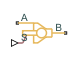# Poppet Valve (TL)

Poppet flow control valve in a thermal liquid network

•Libraries:
Simscape / Fluids / Thermal Liquid / Valves & Orifices / Flow Control Valves

## Description

The Poppet Valve (TL) block represents the flow control within a thermal liquid network. You can specify the seat geometry as either sharp-edged or conical. Set the poppet displacement with the physical signal at port S.### Mass Balance

The mass conservation equation in the valve is

`${\stackrel{˙}{m}}_{A}+{\stackrel{˙}{m}}_{B}=0,$`

where:

• ${\stackrel{˙}{m}}_{A}$ is the mass flow rate into the valve through port A.

• ${\stackrel{˙}{m}}_{B}$ is the mass flow rate into the valve through port B.

### Momentum Balance

The pressure differential over the valve is:

`${p}_{A}-{p}_{B}=\frac{\stackrel{˙}{m}\sqrt{{\stackrel{˙}{m}}^{2}+{\stackrel{˙}{m}}_{cr}^{2}}}{2{\rho }_{Avg}{C}_{d}^{2}{S}^{2}}\left[1-{\left(\frac{{S}_{R}}{S}\right)}^{2}\right]P{R}_{Loss},$`

where:

• pA is the pressure at port A.

• pB is the pressure at port B.

• $\stackrel{˙}{m}$ is the mass flow rate.

• ρAvg is the average liquid density.

• Cd is the value of the Discharge coefficient parameter.

• ${\stackrel{˙}{m}}_{cr}$ is the critical mass flow rate:

`${\stackrel{˙}{m}}_{cr}={\mathrm{Re}}_{cr}{\mu }_{Avg}\sqrt{\frac{\pi }{4}{S}_{R}}.$`

where:

• Recr is the value of the Critical Reynolds number parameter.

• μAvg is the average fluid dynamic viscosity.

• S is the value of the Cross-sectional area at port A and B parameter.

• PRLoss is the pressure ratio:

`$P{R}_{Loss}=\frac{\sqrt{1-{\left({S}_{R}/S\right)}^{2}\left(1-{C}_{d}^{2}\right)}-{C}_{d}\left({S}_{R}/S\right)}{\sqrt{1-{\left({S}_{R}/S\right)}^{2}\left(1-{C}_{d}^{2}\right)}+{C}_{d}\left({S}_{R}/S\right)}.$`

### Energy Balance

The energy conservation equation in the valve is

`${\varphi }_{A}+{\varphi }_{B}=0,$`

where:

• ϕA is the energy flow rate into the valve through port A.

• ϕB is the energy flow rate into the valve through port B.

### Opening Area With a Sharp-Edged Seat

When you set to `Sharp-edged`, the block calculates the valve opening area using

`$A=\pi {r}_{o}\left(1-{\left(\frac{{r}_{p}}{{d}_{OB}}\right)}^{2}\right){d}_{OB}\left(h\right)+{A}_{leak},$`

where:

• ro is the valve orifice radius.

• rp is the valve poppet radius.

• dOB(h) is the distance between the center of the poppet and the edge of the orifice. This distance is a function of the valve lift,h.

• Aleak is the leakage area.

The maximum displacement, hmax, is

`${h}_{\mathrm{max}}=\sqrt{\frac{2{r}_{p}^{2}-{r}_{o}^{2}+{r}_{o}\sqrt{{r}_{o}^{2}+4{r}_{p}^{2}}}{2}}-\sqrt{{r}_{p}^{2}-{r}_{o}^{2}}.$`

The figure shows a schematic of a hard-edged seat.### Opening Area With a Conical Seat

When you set to `Conical`, the block calculates the valve opening area using a geometric relationship, such that

`$A=\pi {r}_{p}\mathrm{sin}\left(\theta \right)h+\frac{\pi }{2}\mathrm{sin}\left(\frac{\theta }{2}\right)\mathrm{sin}\left(\theta \right){h}^{2}+{A}_{leak}.$`

The maximum displacement, hmax, is:

`${h}_{\mathrm{max}}=\frac{\sqrt{{r}_{p}^{2}+\frac{{r}_{o}^{2}}{\mathrm{cos}\left(\frac{\theta }{2}\right)}}-{r}_{p}}{\mathrm{sin}\left(\frac{\theta }{2}\right)},$`

where θ is the Cone angle. The figure shows a schematic of a conical seat.### Numerically Smoothed Displacement

The block calculates the poppet displacement, h, such that

`$h=\left\{\begin{array}{ll}0,\hfill & \left(S-{S}_{\mathrm{min}}\right)\le 0\hfill \\ {h}_{Max},\hfill & \left(S-{S}_{\mathrm{min}}\right)\ge {h}_{Max}\hfill \\ \left(S-{S}_{\mathrm{min}}\right),\hfill & \text{Else}\hfill \end{array}$`

where:

• S is the physical signal input.

• Smin is the Poppet position when in the seat parameter.

• hMax is the maximum displacement.

If the parameter is nonzero, the block smoothly saturates the poppet displacement between `0` and hMax.

## Ports

### Conserving

expand all

Thermal liquid conserving port associated with valve inlet A.

Thermal liquid conserving port associated with valve inlet B.

### Input

expand all

Physical signal port associated with the displacement of the poppet, in m.

## Parameters

expand all

Geometry of the valve seat. The block uses this parameter to calculate the open area between the poppet and seat.

Angle of the seat opening.

#### Dependencies

To enable this parameter, set Valve seat specification to `Conical`.

Diameter of the poppet.

Seat orifice diameter.

Poppet offset when valve is shut. A positive, nonzero value indicates a partially open valve. A negative, nonzero value indicates an overlapped valve that remains shut for an initial displacement set by the physical signal at port .

Sum of all gaps when the valve is in the fully shut position. The block saturates smaller numbers to this value. This parameter contributes to numerical stability by maintaining continuity in the flow.

Continuous smoothing factor that introduces a layer of gradual change to the flow response when the valve is in the near-open or near-shut positions. Set this value to a nonzero number less than one to increase the stability of your simulation in these regimes.

Area at ports A and B, which are used in the pressure-flow rate equation that determines the mass flow rate through the valve.

Correction factor that accounts for discharge losses in theoretical flows.

Upper Reynolds number limit for laminar flow through the orifice.

## Version History

Introduced in R2016a